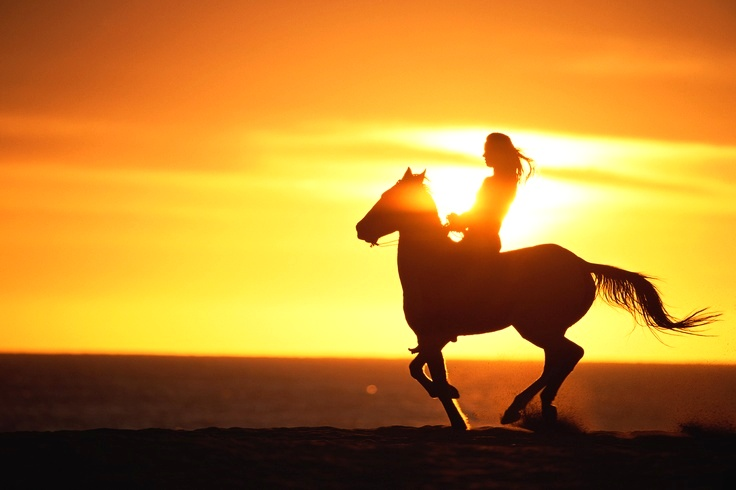Home » How Do Odds Work in Betting on Horses?

# How Do Odds Work in Betting on Horses?Betting on horse races can be an exciting and rewarding activity, but it can also be a bit intimidating if you don’t understand the odds. Understanding the odds is a crucial part of betting on horse races, and it’s important to understand what they mean and how they are calculated. This article will provide an overview of how odds work in betting on horses and how to read them.

## What Are Horse Racing Odds?

Horse racing odds are the numbers used to represent the chances of a horse winning a race. They are expressed as fractions or decimals and indicate the likelihood of a horse winning the race. Odds can be displayed in different formats, such as fractional, decimal, or American.

## Fractional Odds

Fractional odds are the most commonly used format for horse racing. They are expressed as a fraction, such as 10/1 or 5/2. The first figure in the fraction is the amount you would win if your horse won the race. The second figure is the amount you would have to bet in order to win that amount. For example, a fractional odds of 10/1 means that if you bet \$1 and your horse wins, you would win \$10.

## Decimal Odds

Decimal odds are becoming increasingly popular for horse racing. They are expressed as a decimal number, such as 5.00 or 2.50. The number represents the amount you will win for every dollar wagered. For example, a decimal odds of 5.00 means that if you bet \$1 and your horse wins, you will win \$5.

## American Odds

American odds are used mainly in the United States. They are expressed as a positive or negative number, such as +400 or -200. Positive numbers indicate how much you will win for every \$100 wagered. For example, a positive American odds of +400 means that if you bet \$100 and your horse wins, you will win \$400. Negative numbers indicate how much you must bet in order to win \$100. For example, a negative American odds of -200 means that if you bet \$200 and your horse wins, you will win \$100.

Related content  What Kind of Horse Did the US Cavalry Use?

## Calculating the Odds

The odds for a horse race are calculated by taking into account the horse’s past performance, the condition of the track, the weather, and other factors. The odds are determined by the bookmakers, who take into account all of the relevant information and set the odds accordingly.

## Understanding the Odds

When betting on horses, it’s important to understand the odds. The higher the odds, the less likely it is for the horse to win. Conversely, the lower the odds, the more likely it is for the horse to win. It’s important to understand that the odds do not guarantee that a horse will win, but they do provide an indication of a horse’s chances.